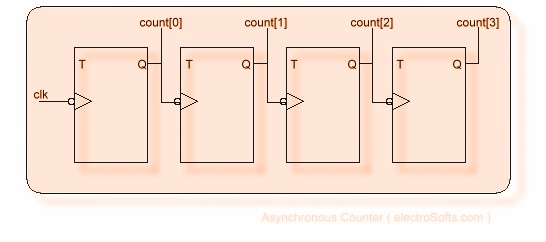## 2014年6月3日 星期二

### ASYNCHRONOUS COUNTER:

ASYNCHRONOUS COUNTER:
Consider a 4-bit asynchronous counter; block diagram using flip-flops is as follows. This is a simple counter without reset or load options.Now look at this code in Verilog.

 module counter( clk, count );input clk;output[3:0] count;reg[3:0] count;wire clk;initial    count = 4'b0;always @( negedge clk )    count <= ~count;always @( negedge count )    count <= ~count;always @( negedge count )    count <= ~count;always @( negedge count )    count <= ~count;endmodule

Keep following points when you select a reg or wire.
• Left hand side of a continuous assignment statement should be a wire. (Signal will always be connected, no need to store it.)
• Left hand side of assignment operations should have a reg(or similar kinds like integer) type of signal. (Value need to be retained in the signal here.)
• All the inputs to a module should be wire.
• Output of a module can be of any type.
• All inout signals should be wire in both the sides of port. (See the diagram bellow: module in is instantiated inside module out.)# initial block executes when simulation starts. Inside an initial block there may be one or more statements. If there is only one statement, we can write it as follows:

 ```initial count = 4’b0;```

If there are more than statements are there to execute, we can use begin.....end or fork.....join statements to combine them.
Within begin....end, statements will executes one after another sequentially. Within fork...join, all the statements will executes simultaneously. More details about begin-end and fork-join you will learn in next chapter.
 ```initial begin a = 1; c = a; end // Both a and c will become 1.```

Initial block may contain if conditions, loops, assignment statements etc., but not continuous assignments. You can have any number of initial blocks in a module. All initial blocks execute simultaneously.
Initial blocks are very useful in testbenches. Consider a code for counter. Actually in a chip, initially counter will have a unpredicted value. But after sometimes, value will increase to its maximum value, where all the bits are zero. Then it will start counting through 0 to maximum value. But in the simulation, initially value will be 'x', and if we don't initialize, it will remain 'x' only. So, we can use initial blocks to initialize all the signals to a valid value.

Always Block:
Always blocks execute always. We can use delay or sensitivity list to control the execution of always block. Syntax is as follows:
always @ ( sencitivity_list )
statements;
Sensitivity list may contain signal names as follows:

always @ ( signal1 or signal2 )
statement;
Above statement will execute whenever signal1 or signal2 changes. we can have 1 to any number of signals in sensitivity list.

always @ ( posedge signal )
statement;
Above statement executes at the positive edge of signal. Similarly we can use negedge for negative edge of the signal.
Without sensitivity list, always block will look like this:
always
#5 clk = ~clk;
This will toggle the signal clk for every 5 nanoseconds.
More about always block, you will learn after studying some examples.

Blocking and Non-blocking Assignments:
If a set of statements has to be executed one after another, we have to use blocking statements. Commonly, we will use blocking statements in combinational logics. Blocking assignments are done using '=', as in a = b;.
Nonblocking assignments are commonly used to implement sequential logic. Non blocking statements without delay in between will execute simultaneously. If you use non-blocking assignments, expressions will be evaluated depending on the delay added in-between and will be assigned simultaneously. Consider these code:

 ```always @( posedge clk ) begin a <= b; b <= a; end /* Value of a and b will be swapped. Commonly used in sequential logics */``` always@ ( b )begin     a = b;     b = a;end/*Value of b will be copied to both a and b. Commonly used in combinational logic*/

 ```module counter_tb; reg clk; wire[3:0] count; counter my_counter( .clk(clk), .count( count ) ); initial begin clk = 0; #200 \$finish; end always begin #2 clk = ~clk; end always @( posedge clk) \$display("Count = %b", count ); endmodule```